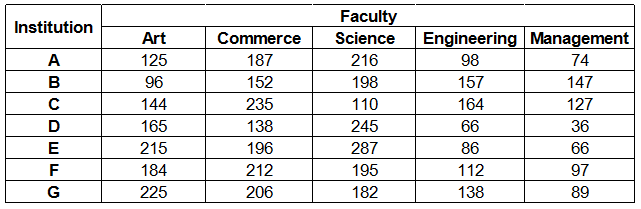### IBPS Clerk 2018 Question 20

Instructions

Study the following table carefully and answer the questions given below :
Number of students studying in different faculties in Seven institutionsQuestion 20

# In which institution, the percentage of students studying Commerce to the total students of that institution is maximum ?

Solution

Total number of students in institutes :

A = 125 + 187 + 216 + 98 + 74 = 700

B = 96 + 152 + 198 + 157 + 147 = 750

C = 144 + 235 + 110 + 164 + 127 = 780

D = 165 + 138 + 245 + 66 + 36 = 650

E = 215 + 196 + 287 + 86 + 66 = 850

F = 184 + 212 + 195 + 112 + 97 = 800

G = 225 + 206 + 182 + 138 + 89 = 840

Now, Percentage of students studying Commerce to the total students of institution :

A = $$\frac{187}{700} \times 100$$ = 26.7 %

B = $$\frac{152}{750} \times 100$$ = 20.2 %

C = $$\frac{235}{780} \times 100$$ = 30.1 %  [MAX]

D = $$\frac{138}{650} \times 100$$ = 21.2 %

E = $$\frac{196}{850} \times 100$$ = 23.1 %

F = $$\frac{212}{800} \times 100$$ = 26.5 %

G = $$\frac{206}{840} \times 100$$ = 24.5 %